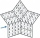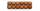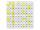# Divisibility + natural numbers - math problems

#### Number of problems found: 127

• Divisibility 2How many divisors have integer number 13?
• DivisibilityDetermine all divisors of number 84.
• All pairsDetermine all pairs (m, n) of natural numbers for which is true: m s (n) = n s (m) = 70, where s (a) denotes the digit sum of the natural number a.
• Count of rootsHow many solutions has equation x. y = 7757 with two unknowns on the set of natural numbers?
• Three-digit integersHow many three-digit natural numbers exist that do not contain zero and are divisible by five?
• Divisible by nineHow many three-digit natural numbers in total are divisible without a remainder by the number 9?
• Twenty-fiveHow many are three-digit natural numbers divisible by 25?
• Sum of the digitsHow many are two-digit natural numbers that have the sum of the digits 9?
• RectanglesHow many rectangles with area 8713 cm2 whose sides are natural numbers are?
• Digit sumFind the smallest natural number n, the digit sum of which is equal to 37.
• Seven numbersWrite seven 4-digit numbers that are divisible by 3 and at the same time by 4.
• Theorem proveWe want to prove the sentence: If the natural number n is divisible by six, then n is divisible by three. From what assumption we started?
• How many 4How many 4 digit numbers that are divisible by 10 can be formed from the numbers 3, 5, 7, 8, 9, 0 such that no number repeats?
• Reminder and quotientThere are given the number C = 281, D = 201. Find the highest natural number S so that the C:S, D:S are with the remainder of 1,
• DivisibilityDetermine the smallest integer which divided 11 gives remainder 4 when divided 15 gives remainder 10 and when divided by 19 gives remainder 16.
• How manyHow many numbers are less than 222 with a digit sum is 8?
• Odd numbersThe sum of four consecutive odd numbers is 1048. Find those numbers ...
• Primes 2Which prime numbers is number 2025 divisible?
• Year 2018The product of the three positive numbers is 2018. What are the numbers?
• Three digits numberFrom the numbers 1, 2, 3, 4, 5 create three-digit numbers that digits not repeat and number is divisible by 2. How many numbers are there?

Do you have an interesting mathematical word problem that you can't solve it? Submit a math problem, and we can try to solve it.

We will send a solution to your e-mail address. Solved examples are also published here. Please enter the e-mail correctly and check whether you don't have a full mailbox.

Please do not submit problems from current active competitions such as Mathematical Olympiad, correspondence seminars etc...

Divisibility - math problems. Natural numbers - math problems.